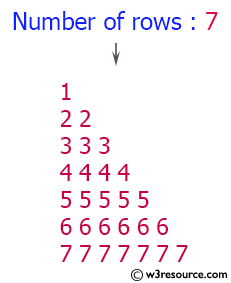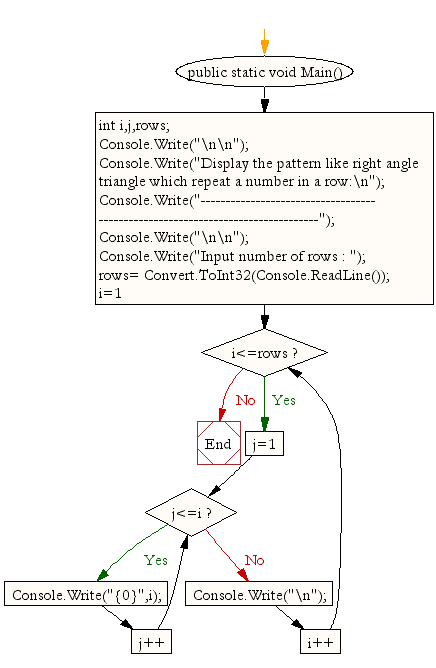﻿ C# - Pattern as a right angle triangle that repeats a number# C# Sharp Exercises: Display the pattern like right angle triangle which repeat a number in a row

## C# Sharp For Loop: Exercise-11 with Solution

Write a program in C# Sharp to make such a pattern like right angle triangle with a number which will repeat a number in a row.
The pattern is as follows:

```1
22
333
4444
```

Pictorial Presentation:Sample Solution:-

C# Sharp Code:

``````using System;
public class Exercise11
{
public static void Main()
{
int i,j,rows;

Console.Write("\n\n");
Console.Write("Display the pattern like right angle triangle which repeat a number in a row:\n");
Console.Write("-------------------------------------------------------------------------------");
Console.Write("\n\n");

Console.Write("Input number of rows : ");
for(i=1;i<=rows;i++)
{
for(j=1;j<=i;j++)
Console.Write("{0}",i);
Console.Write("\n");
}
}
}
```
```

Sample Output:

```Display the pattern like right angle triangle which repeat a number in a row:
-------------------------------------------------------------------------------

Input number of rows : 7
1
22
333
4444
55555
666666
7777777
```

Flowchart:C# Sharp Code Editor:

Contribute your code and comments through Disqus.

What is the difficulty level of this exercise?

Test your Programming skills with w3resource's quiz.

﻿